# Image Warp

This example shows how to implement affine and projective transforms for FPGAs.

Image warping is a common technique in image processing and computer graphics. This technique generates an image to specified requirements by geometrically distorting an input image, an approach that is closely related to the morphing technique. Image warping has diverse applications, such as registration in remote sensing and creating visual special effects in the entertainment industry.

The warp algorithm maps locations in the output image to corresponding locations in the input image, a process known as inverse mapping. The hardware-friendly warp implementation in this example performs the same operation as imwarp (Image Processing Toolbox) function.

The algorithm in this example performs an inverse geometric transform and calculates the output pixel intensities by using bilinear interpolation. This implementation does not require external DDR memory and instead resamples the output pixel intensities by using the on-chip BRAM memory.

### Image Warp Algorithm

The image warping algorithm maps the pixel locations of the output warped image to the pixel locations in the input image by using a reverse mapping technique. This diagram shows the stages of the algorithm.Compute Transformation: This stage computes the inverse transformation matrix. The calculated transformation parameters include the output bounds and the transformation matrix tForm. The algorithm requires these bounds to compute the integer pixel coordinates of the output image. The algorithm requires tForm to transform the integer pixel coordinates in the output image to the corresponding coordinates of the input image.

Inverse Geometric Transform: An inverse geometric transformation translates a point in one image plane onto another image plane. In image warping, this operation maps the integer pixel coordinates in the output image to the corresponding coordinates of the input image by using the transformation matrix. If (u,v) is an integer pixel coordinate in the warped output image and (x,y) is the corresponding coordinate of the input image, then this equation describes the transformation.tForm is the inverse transformation matrix. To convert from homogeneous to cartesian coordinates,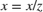and.

Bilinear Interpolation: The warping algorithm can produce coordinates (x,y) with noninteger values. To generate the pixel intensity values at each integer position, a warp algorithm can use various resampling techniques. The example uses bilinear interpolation. Interpolation resamples the image intensity values corresponding to the generated coordinates.

### HDL Implementation

The figure shows the top-level view of the ImageWarpHDL model. The Input Image block imports the images from files. The Frame To Pixels block converts the input image frames to a pixel stream and a pixelcontrol bus as inputs to the ImageWarpHDLALgorithm subsystem. This subsystem takes these mask parameters.

• Number of input lines to buffer — The provided ComputeImageWarpCacheOffset function calculates this parameter from the transformation matrix.

• Input active pixels — Horizontal size of the input image.

• Input active lines — Vertical size of the input image.

The ImageWarpHDLAlgorithm subsystem warps the input image as specified by the value of the tForm input port. The Pixels To Frame block converts the streams of output pixels back to frames. The ImageViewer subsystem displays the input frame and the corresponding warped output.

open_system('ImageWarpHDL'); set(allchild(0),'Visible','off');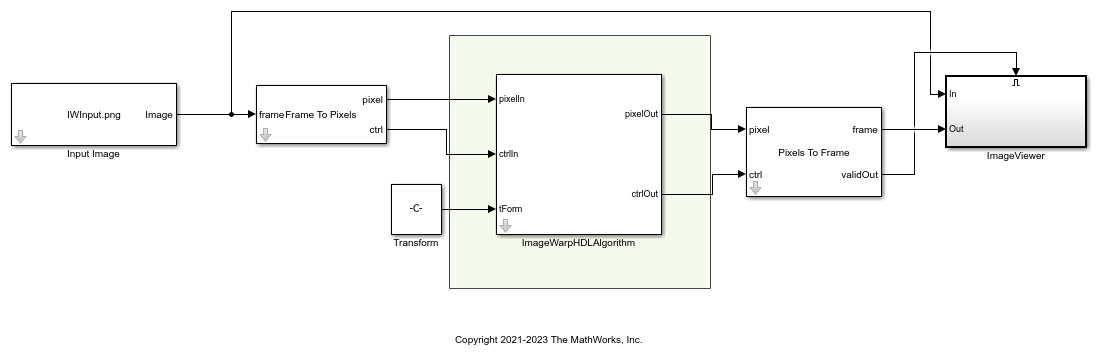The InitFcn callback function loads the transformation matrix from tForm.mat. Alternatively, you can generate your own transformation matrix (in the form of a nine-element column vector) and use this vector as the input to the ImageWarpHDLAlgorithm subsystem. The InitFcn callback function of the example model also computes the cache offset by calling the ComputeImageWarpCacheOffset function. This function calculates the offset and displacement parameters of the output image from the transformation matrix and output image dimensions.

In the ImageWarpHDLAlgorithm subsystem, the GenerateControl subsystem uses the displacement parameter to modify the pixelcontrol bus from the input ctrl port. The CoordinateGeneration subsystem generates the row and column pixel coordinates (u,v) of the output image by using two HDL counters. The InverseTransform subsystem maps these coordinates onto their corresponding coordinates (x,y) of the input image.

The AddressGeneration subsystem calculates the addresses of the four neighbors of (x,y) required for interpolation. This subsystem also computes the parameters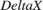,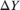,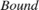, and, which the model uses for bilinear interpolation.

The Interpolation subsystem stores the pixel intensities of the input image in a memory. To calculate each output pixel intensity, the subsystem reads the four neighbor pixel values and computes their weighted sum.

open_system('ImageWarpHDL/ImageWarpHDLAlgorithm','force');### Inverse Transformation

The HDL implementation of the inverse geometric transformation multiplies the coordinates [u v 1] by the transformation matrix. The Transformation subsystem implements the matrix multiplication with Product blocks, which multiply the integer coordinates of the output image by each element of the transformation matrix. For this operation, the Transformation subsystem splits the transformation matrix into individual elements by using a Demux block. The HomogeneousToCartesian subsystem converts the generated homogeneous coordinates, [x y z] back to the cartesian format [x y] for further processing. The HomogeneousToCartesian subsystem uses a Reciprocal block configured to use the ShiftAdd architecture, and the Product blocks that compute x and y use the ShiftAdd architecture for better hardware clock speed. To see these parameters, right-click the block and select HDL Code > HDL Block Properties.

open_system('ImageWarpHDL/ImageWarpHDLAlgorithm/InverseTransform','force');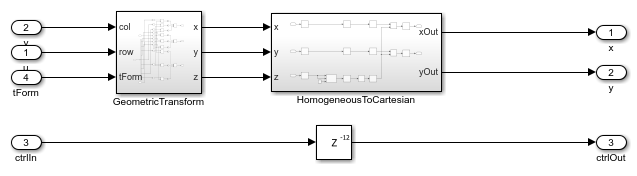The AddressGeneration subsystem calculates the displacement of each pixel from its neighboring pixels by using the mapped coordinate (x,y) of the input raw image. The subsystem also rounds the coordinates to the nearest integer toward negative infinity.

open_system('ImageWarpHDL/ImageWarpHDLAlgorithm/AddressGeneration','force');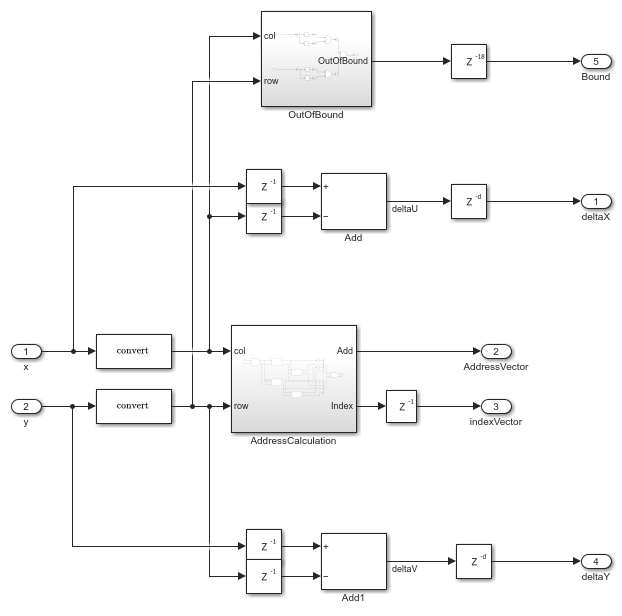The AddressCalculation subsystem checks the coordinates against the bounds of the input images. If any coordinate is outside the image dimensions, the subsystem sets that coordinate to the boundary value. Next, the subsystem calculates the index of the address for each of the four neighborhood pixels in the CacheMemory subsystem. The index represents the column of the cache. The subsystem finds the index for each address by using the even and odd nature of the incoming column and row coordinates, as determined by the Extract Bits block.

% ========================== % |Row || Col || Index || % ========================== % |Odd || Odd || 1 || % |Even || Odd || 2 || % |Odd || Even || 3 || % |Even || Even || 4 || % ========================== 

This equation specifies the address of the neighborhood pixels.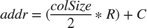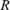is the row coordinate and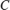is the column coordinate. When row is even, then. When row is odd, then. When col is even, then. When col is odd, then.

The IndexChangeForMemoryAccess MATLAB Function block in the AddressCalculation subsystem rearranges the addresses in increasing order of their indices. This operation ensures the correct fetching of data from the CacheMemory block. This subsystem passes the addresses to the CacheMemory subsystem, and passes Index,, andto the Interpolation subsystem.

The OutOfBound subsystem checks whether the (x,y) coordinates are out of bounds (that is, if any coordinate is outside the image dimensions). If the coordinate is out of bounds, the subsystem sets the corresponding output pixel to an intensity value of 0.

Finally, a Vector Concatenate block creates vectors of the addresses and indices.

### Interpolation

The Interpolation subsystem is a For Each block, which replicates its operation depending on the dimensions of the input pixel. For example, if the input is an RGB image, then the input pixel dimensions are 1-by-3, and the model includes three instances of this operation. Because the model uses a For Each block, it supports RGB or grayscale input. The operation inside the Interpolation subsystem comprises two subsystems: BilinearInterpolation and CacheMemory.

open_system('ImageWarpHDL/ImageWarpHDLAlgorithm/Interpolation','force');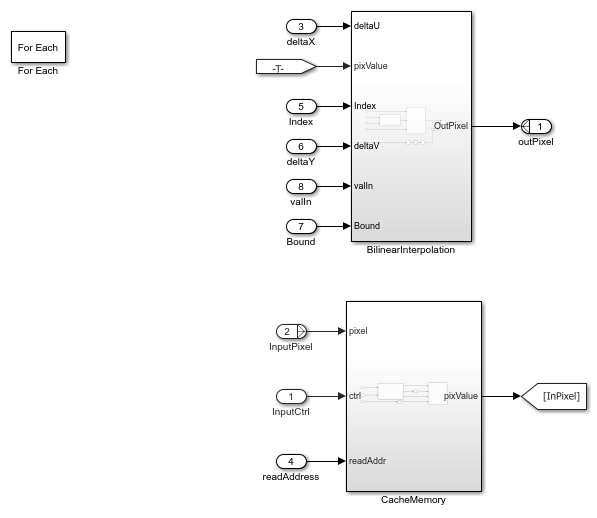### Cache Memory

The CacheMemory subsystem contains a Simple Dual Port RAM block. The subsystem buffers the input pixels to form [Line 1 Pixel 1 | Line 2 Pixel 1 | Line 1 Pixel 2 | Line 2 Pixel 2] in the RAM. By using this configuration, the algorithm can read all four neighboring pixels in one cycle. The example calculates the required size of the cache memory from the offset output of the ComputeImageWarpCacheOffset function. The offset is the sum of the maximum deviation and the first row map. The first row map is the maximum value of the input image row coordinate that corresponds to the first row of the output undistorted image. The maximum deviation is the greatest difference between the maximum and minimum row coordinates for each row of the input image row map.

The WriteControl subsystem forms vectors of incoming pixels, write enables, and write addresses. The AddressGeneration subsystem provides a vector of read addresses. The vector of pixels from the RAM is the input to the BilinearInterpolation subsystem.

open_system('ImageWarpHDL/ImageWarpHDLAlgorithm/Interpolation/CacheMemory','force');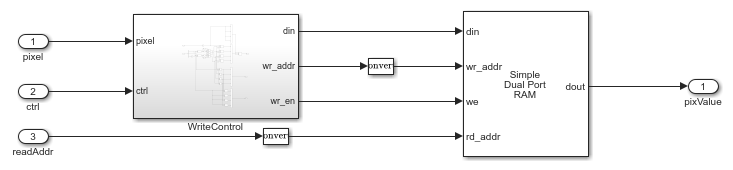### Bilinear Interpolation

The BilinearInterpolation subsystem rearranges the vector of read pixels from the cache to their original indices. Then, the BilinearInterpolationEquation subsystem calculates a weighted sum of the neighborhood pixels. The result of the interpolation is the value of the output warped pixel.

open_system('ImageWarpHDL/ImageWarpHDLAlgorithm/Interpolation/BilinearInterpolation','force');In the equation and the diagram, (u,v) is the coordinate of the input pixel generated by the inverse tranformation stage.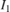,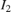,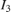, andare the four neighboring pixels, and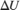and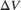are the displacements of the target pixel from its neighboring pixels. This stage of the algorithm computes the weighted average of the four neighboring pixels by using this equation.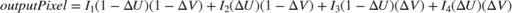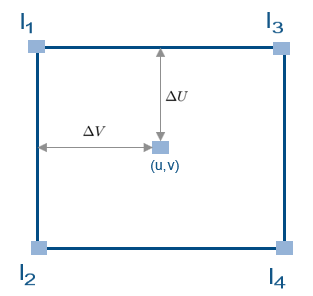### Simulation and Results

This example uses a 480p RGB input image. The input pixels use the uint8 data type for either grayscale and RGB input images.

This implementation uses on chip BRAM memory rather than external DDR memory. The amount of BRAM required for the computation of output pixel intensities is directly proportional to the number of input lines required to be buffered in the cache. This bar graph shows the number of lines required in the cache for different angles of rotation of the output image. For this graph, the scaling factor is 1.1, and the translation in the x- and y-directions is 0.6 and 0.3, respectively.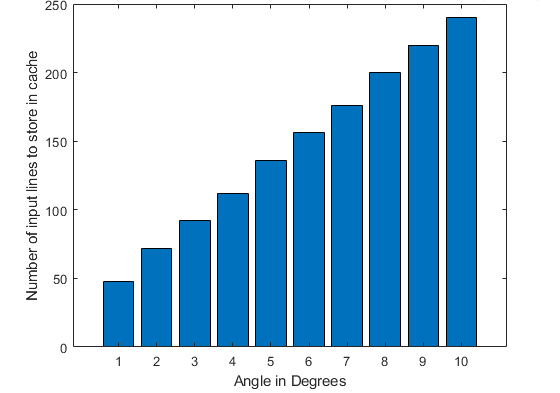This figure shows the input image and the corresponding output image rotated by an angle of four degrees, scaled by a factor of 1.1, and translated by 0.4 and 0.8 in the x- and y-directions, respectively. The results of the ImageWarpHDL model match the output of the imwarp function in MATLAB.To check and generate the HDL code referenced in this example, you must have an HDL Coder™ license.

To generate the HDL code, use this command.

makehdl('ImageWarpHDL/ImageWarpHDLAlgorithm') 

To generate the test bench, use this command.

makehdltb('ImageWarpHDL/ImageWarpHDLAlgorithm') 

This design was synthesized using Xilinx® Vivado® for the Xilinx® Zynq®-7000 SoC ZC706 development kit and met a timing requirement of over 200MHz. The table shows the resource utilization for the HDL subsystem.

% =============================================================== % |Model Name || ImageWarpHDL || % =============================================================== % |Input Image Resolution || 480 x 640 || % |Slice LUTs || 7325 || % |Slice Registers || 7431 || % |BRAM || 97 || % |Total DSP Blocks || 82 || % ===============================================================